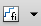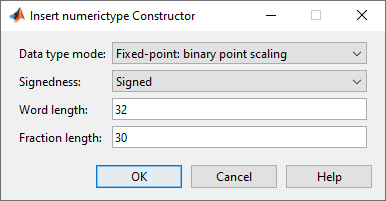numerictype Object Construction

numerictype Object Syntaxes

numerictype objects define the data type and scaling attributes of fi objects, as well as Simulink® signals and model parameters. You can create numerictype objects in Fixed-Point Designer™ software in one of two ways:

• You can use the numerictype constructor function to create a new object.

• You can use the numerictype constructor function to copy an existing numerictype object.

To create a default numerictype object, type

T = numerictype
T =

DataTypeMode: Fixed-point: binary point scaling
Signedness: Signed
WordLength: 16
FractionLength: 15

To see all of the numerictype object syntaxes, refer to the numerictype constructor function reference page.

The following examples show different ways of constructing numerictype objects. For more examples of constructing numerictype objects, see the Examples on the numerictype constructor function reference page.

Example: Construct a numerictype Object with Property Name and Property Value Pairs

When you create a numerictype object using property name and property value pairs, Fixed-Point Designer software first creates a default numerictype object, and then, for each property name you specify in the constructor, assigns the corresponding value.

This behavior differs from the behavior that occurs when you use a syntax such as T = numerictype(s,w), where you only specify the property values in the constructor. Using such a syntax results in no default numerictype object being created, and the numerictype object receives only the assigned property values that are specified in the constructor.

The following example shows how the property name/property value syntax creates a slightly different numerictype object than the property values syntax, even when you specify the same property values in both constructors.

To demonstrate this difference, suppose you want to create an unsigned numerictype object with a word length of 32 bits.

First, create the numerictype object using property name/property value pairs.

T1 = numerictype('Signed',0,'WordLength',32)
T1 =

DataTypeMode: Fixed-point: binary point scaling
Signedness: Unsigned
WordLength: 32
FractionLength: 15

The numerictype object T1 has the same DataTypeMode and FractionLength as a default numerictype object, but the WordLength and Signed properties are overwritten with the values you specified.

Now, create another unsigned 32 bit numerictype object, but this time specify only property values in the constructor.

T2 = numerictype(0,32)
T2 =

DataTypeMode: Fixed-point: unspecified scaling
Signedness: Unsigned
WordLength: 32

Unlike T1, T2 only has the property values you specified. The DataTypeMode of T2 is Fixed-Point: unspecified scaling, so no fraction length is assigned.

fi objects cannot have unspecified numerictype properties. Thus, all unspecified numerictype object properties become specified at the time of fi object creation.

Example: Copy a numerictype Object

To copy a numerictype object, use assignment:

T = numerictype;
U = T;
isequal(T,U)
ans =

logical

1

Example: Build numerictype Object Constructors in a GUI

When you are working with files in MATLAB®, you can build your numerictype object constructors using the Insert numerictype Constructor dialog box. After specifying the properties of the numerictype object in the dialog box, you can insert the pre-populated numerictype object constructor at a specific location in your file.

For example, create a signed numerictype object with binary-point scaling, a word length of 32 bits and a fraction length of 30 bits:

1. On the Home tab, in the File section, click New > Script to open the MATLAB Editor

2. On the Editor tab, in the Edit section of the toolstrip, clickin the Insert button group. Click the Insert numerictype to open the Insert numerictype Constructor dialog box.

3. Use the edit boxes and drop-down menus to specify the following properties of the numerictype object:

• Data type mode: Fixed-point: binary point scaling

• Signedness: Signed

• Word length: 32

• Fraction length: 304. To insert the numerictype object constructor in your file, place your cursor at the desired location in the file, and click on the Insert numerictype Constructor dialog box. Clicking closes the Insert numerictype Constructor dialog box and automatically populates the numerictype object constructor in your file:

numerictype(1, 32, 30)

Fixed-Point Designer DocumentationGet trial now